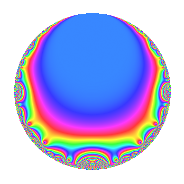# Properties

 Label 4002.2.a.xLevel 4002 Weight 2 Character orbit 4002.a Self dual Yes Analytic conductor 31.956 Analytic rank 0 Dimension 2 CM No Inner twists 1

# Related objects

## Newspace parameters

 Level: $$N$$ = $$4002 = 2 \cdot 3 \cdot 23 \cdot 29$$ Weight: $$k$$ = $$2$$ Character orbit: $$[\chi]$$ = 4002.a (trivial)

## Newform invariants

 Self dual: Yes Analytic conductor: $$31.9561308889$$ Analytic rank: $$0$$ Dimension: $$2$$ Coefficient field: $$\Q(\sqrt{6})$$ Coefficient ring: $$\Z[a_1, \ldots, a_{7}]$$ Coefficient ring index: $$1$$ Fricke sign: $$-1$$ Sato-Tate group: $\mathrm{SU}(2)$

## $q$-expansion

Coefficients of the $$q$$-expansion are expressed in terms of $$\beta = \sqrt{6}$$. We also show the integral $$q$$-expansion of the trace form.

 $$f(q)$$ $$=$$ $$q$$ $$+ q^{2}$$ $$+ q^{3}$$ $$+ q^{4}$$ $$+ 4 q^{5}$$ $$+ q^{6}$$ $$+ ( 2 - \beta ) q^{7}$$ $$+ q^{8}$$ $$+ q^{9}$$ $$+O(q^{10})$$ $$q$$ $$+ q^{2}$$ $$+ q^{3}$$ $$+ q^{4}$$ $$+ 4 q^{5}$$ $$+ q^{6}$$ $$+ ( 2 - \beta ) q^{7}$$ $$+ q^{8}$$ $$+ q^{9}$$ $$+ 4 q^{10}$$ $$-2 \beta q^{11}$$ $$+ q^{12}$$ $$+ ( -2 + 2 \beta ) q^{13}$$ $$+ ( 2 - \beta ) q^{14}$$ $$+ 4 q^{15}$$ $$+ q^{16}$$ $$+ 2 \beta q^{17}$$ $$+ q^{18}$$ $$+ ( -2 + \beta ) q^{19}$$ $$+ 4 q^{20}$$ $$+ ( 2 - \beta ) q^{21}$$ $$-2 \beta q^{22}$$ $$- q^{23}$$ $$+ q^{24}$$ $$+ 11 q^{25}$$ $$+ ( -2 + 2 \beta ) q^{26}$$ $$+ q^{27}$$ $$+ ( 2 - \beta ) q^{28}$$ $$+ q^{29}$$ $$+ 4 q^{30}$$ $$-6 q^{31}$$ $$+ q^{32}$$ $$-2 \beta q^{33}$$ $$+ 2 \beta q^{34}$$ $$+ ( 8 - 4 \beta ) q^{35}$$ $$+ q^{36}$$ $$-2 q^{37}$$ $$+ ( -2 + \beta ) q^{38}$$ $$+ ( -2 + 2 \beta ) q^{39}$$ $$+ 4 q^{40}$$ $$+ ( -4 + 2 \beta ) q^{41}$$ $$+ ( 2 - \beta ) q^{42}$$ $$+ ( 10 - \beta ) q^{43}$$ $$-2 \beta q^{44}$$ $$+ 4 q^{45}$$ $$- q^{46}$$ $$+ 4 q^{47}$$ $$+ q^{48}$$ $$+ ( 3 - 4 \beta ) q^{49}$$ $$+ 11 q^{50}$$ $$+ 2 \beta q^{51}$$ $$+ ( -2 + 2 \beta ) q^{52}$$ $$+ ( -4 + 2 \beta ) q^{53}$$ $$+ q^{54}$$ $$-8 \beta q^{55}$$ $$+ ( 2 - \beta ) q^{56}$$ $$+ ( -2 + \beta ) q^{57}$$ $$+ q^{58}$$ $$+ 2 \beta q^{59}$$ $$+ 4 q^{60}$$ $$+ ( -2 - 2 \beta ) q^{61}$$ $$-6 q^{62}$$ $$+ ( 2 - \beta ) q^{63}$$ $$+ q^{64}$$ $$+ ( -8 + 8 \beta ) q^{65}$$ $$-2 \beta q^{66}$$ $$+ 2 q^{67}$$ $$+ 2 \beta q^{68}$$ $$- q^{69}$$ $$+ ( 8 - 4 \beta ) q^{70}$$ $$+ ( -6 + 2 \beta ) q^{71}$$ $$+ q^{72}$$ $$+ ( -2 + 2 \beta ) q^{73}$$ $$-2 q^{74}$$ $$+ 11 q^{75}$$ $$+ ( -2 + \beta ) q^{76}$$ $$+ ( 12 - 4 \beta ) q^{77}$$ $$+ ( -2 + 2 \beta ) q^{78}$$ $$+ 10 q^{79}$$ $$+ 4 q^{80}$$ $$+ q^{81}$$ $$+ ( -4 + 2 \beta ) q^{82}$$ $$+ ( -4 - \beta ) q^{83}$$ $$+ ( 2 - \beta ) q^{84}$$ $$+ 8 \beta q^{85}$$ $$+ ( 10 - \beta ) q^{86}$$ $$+ q^{87}$$ $$-2 \beta q^{88}$$ $$+ ( 8 - 2 \beta ) q^{89}$$ $$+ 4 q^{90}$$ $$+ ( -16 + 6 \beta ) q^{91}$$ $$- q^{92}$$ $$-6 q^{93}$$ $$+ 4 q^{94}$$ $$+ ( -8 + 4 \beta ) q^{95}$$ $$+ q^{96}$$ $$+ ( -2 + 7 \beta ) q^{97}$$ $$+ ( 3 - 4 \beta ) q^{98}$$ $$-2 \beta q^{99}$$ $$+O(q^{100})$$ $$\operatorname{Tr}(f)(q)$$ $$=$$ $$2q$$ $$\mathstrut +\mathstrut 2q^{2}$$ $$\mathstrut +\mathstrut 2q^{3}$$ $$\mathstrut +\mathstrut 2q^{4}$$ $$\mathstrut +\mathstrut 8q^{5}$$ $$\mathstrut +\mathstrut 2q^{6}$$ $$\mathstrut +\mathstrut 4q^{7}$$ $$\mathstrut +\mathstrut 2q^{8}$$ $$\mathstrut +\mathstrut 2q^{9}$$ $$\mathstrut +\mathstrut O(q^{10})$$ $$2q$$ $$\mathstrut +\mathstrut 2q^{2}$$ $$\mathstrut +\mathstrut 2q^{3}$$ $$\mathstrut +\mathstrut 2q^{4}$$ $$\mathstrut +\mathstrut 8q^{5}$$ $$\mathstrut +\mathstrut 2q^{6}$$ $$\mathstrut +\mathstrut 4q^{7}$$ $$\mathstrut +\mathstrut 2q^{8}$$ $$\mathstrut +\mathstrut 2q^{9}$$ $$\mathstrut +\mathstrut 8q^{10}$$ $$\mathstrut +\mathstrut 2q^{12}$$ $$\mathstrut -\mathstrut 4q^{13}$$ $$\mathstrut +\mathstrut 4q^{14}$$ $$\mathstrut +\mathstrut 8q^{15}$$ $$\mathstrut +\mathstrut 2q^{16}$$ $$\mathstrut +\mathstrut 2q^{18}$$ $$\mathstrut -\mathstrut 4q^{19}$$ $$\mathstrut +\mathstrut 8q^{20}$$ $$\mathstrut +\mathstrut 4q^{21}$$ $$\mathstrut -\mathstrut 2q^{23}$$ $$\mathstrut +\mathstrut 2q^{24}$$ $$\mathstrut +\mathstrut 22q^{25}$$ $$\mathstrut -\mathstrut 4q^{26}$$ $$\mathstrut +\mathstrut 2q^{27}$$ $$\mathstrut +\mathstrut 4q^{28}$$ $$\mathstrut +\mathstrut 2q^{29}$$ $$\mathstrut +\mathstrut 8q^{30}$$ $$\mathstrut -\mathstrut 12q^{31}$$ $$\mathstrut +\mathstrut 2q^{32}$$ $$\mathstrut +\mathstrut 16q^{35}$$ $$\mathstrut +\mathstrut 2q^{36}$$ $$\mathstrut -\mathstrut 4q^{37}$$ $$\mathstrut -\mathstrut 4q^{38}$$ $$\mathstrut -\mathstrut 4q^{39}$$ $$\mathstrut +\mathstrut 8q^{40}$$ $$\mathstrut -\mathstrut 8q^{41}$$ $$\mathstrut +\mathstrut 4q^{42}$$ $$\mathstrut +\mathstrut 20q^{43}$$ $$\mathstrut +\mathstrut 8q^{45}$$ $$\mathstrut -\mathstrut 2q^{46}$$ $$\mathstrut +\mathstrut 8q^{47}$$ $$\mathstrut +\mathstrut 2q^{48}$$ $$\mathstrut +\mathstrut 6q^{49}$$ $$\mathstrut +\mathstrut 22q^{50}$$ $$\mathstrut -\mathstrut 4q^{52}$$ $$\mathstrut -\mathstrut 8q^{53}$$ $$\mathstrut +\mathstrut 2q^{54}$$ $$\mathstrut +\mathstrut 4q^{56}$$ $$\mathstrut -\mathstrut 4q^{57}$$ $$\mathstrut +\mathstrut 2q^{58}$$ $$\mathstrut +\mathstrut 8q^{60}$$ $$\mathstrut -\mathstrut 4q^{61}$$ $$\mathstrut -\mathstrut 12q^{62}$$ $$\mathstrut +\mathstrut 4q^{63}$$ $$\mathstrut +\mathstrut 2q^{64}$$ $$\mathstrut -\mathstrut 16q^{65}$$ $$\mathstrut +\mathstrut 4q^{67}$$ $$\mathstrut -\mathstrut 2q^{69}$$ $$\mathstrut +\mathstrut 16q^{70}$$ $$\mathstrut -\mathstrut 12q^{71}$$ $$\mathstrut +\mathstrut 2q^{72}$$ $$\mathstrut -\mathstrut 4q^{73}$$ $$\mathstrut -\mathstrut 4q^{74}$$ $$\mathstrut +\mathstrut 22q^{75}$$ $$\mathstrut -\mathstrut 4q^{76}$$ $$\mathstrut +\mathstrut 24q^{77}$$ $$\mathstrut -\mathstrut 4q^{78}$$ $$\mathstrut +\mathstrut 20q^{79}$$ $$\mathstrut +\mathstrut 8q^{80}$$ $$\mathstrut +\mathstrut 2q^{81}$$ $$\mathstrut -\mathstrut 8q^{82}$$ $$\mathstrut -\mathstrut 8q^{83}$$ $$\mathstrut +\mathstrut 4q^{84}$$ $$\mathstrut +\mathstrut 20q^{86}$$ $$\mathstrut +\mathstrut 2q^{87}$$ $$\mathstrut +\mathstrut 16q^{89}$$ $$\mathstrut +\mathstrut 8q^{90}$$ $$\mathstrut -\mathstrut 32q^{91}$$ $$\mathstrut -\mathstrut 2q^{92}$$ $$\mathstrut -\mathstrut 12q^{93}$$ $$\mathstrut +\mathstrut 8q^{94}$$ $$\mathstrut -\mathstrut 16q^{95}$$ $$\mathstrut +\mathstrut 2q^{96}$$ $$\mathstrut -\mathstrut 4q^{97}$$ $$\mathstrut +\mathstrut 6q^{98}$$ $$\mathstrut +\mathstrut O(q^{100})$$

## Embeddings

For each embedding $$\iota_m$$ of the coefficient field, the values $$\iota_m(a_n)$$ are shown below.

For more information on an embedded modular form you can click on its label.

Label $$\iota_m(\nu)$$ $$a_{2}$$ $$a_{3}$$ $$a_{4}$$ $$a_{5}$$ $$a_{6}$$ $$a_{7}$$ $$a_{8}$$ $$a_{9}$$ $$a_{10}$$
1.1
 2.44949 −2.44949
1.00000 1.00000 1.00000 4.00000 1.00000 −0.449490 1.00000 1.00000 4.00000
1.2 1.00000 1.00000 1.00000 4.00000 1.00000 4.44949 1.00000 1.00000 4.00000
 $$n$$: e.g. 2-40 or 990-1000 Significant digits: Format: Complex embeddings Normalized embeddings Satake parameters Satake angles

## Inner twists

This newform does not admit any (nontrivial) inner twists.

## Atkin-Lehner signs

$$p$$ Sign
$$2$$ $$-1$$
$$3$$ $$-1$$
$$23$$ $$1$$
$$29$$ $$-1$$

## Hecke kernels

This newform can be constructed as the intersection of the kernels of the following linear operators acting on $$S_{2}^{\mathrm{new}}(\Gamma_0(4002))$$:

 $$T_{5}$$ $$\mathstrut -\mathstrut 4$$ $$T_{7}^{2}$$ $$\mathstrut -\mathstrut 4 T_{7}$$ $$\mathstrut -\mathstrut 2$$ $$T_{11}^{2}$$ $$\mathstrut -\mathstrut 24$$